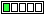All about flooble | fun stuff | Get a free chatterbox | Free JavaScript | Avatarsperplexus dot infoTimes Square 2 (Posted on 2018-10-08)Place a 1-digit number in each of the 16 white cells below so that the product of the numbers in each row will be the number shown to the right of that row, and the product of the numbers in each column will be the number at the bottom of that column.

There will be a digit 1 in exactly one cell in each row and exactly one cell in each column.

 75 28 12 45 50 20 42 27

From Mensa Puzzle Calendar 2018 by Fraser Simpson, Workman Publishing, New York. Puzzle for October 3.

 See The Solution Submitted by Charlie No RatingComments: ( Back to comment list | You must be logged in to post comments.)Analytical Steps (solution) Comment 2 of 2 |start with the first column which has a product of 50=2*5*5 so the only possible way get 50 from the product of 4 single digit number is 1*2*5*5.  Now 75 and 45 are the only rows that are multiples of 5 so the 5's have to go in those rows.

Now look at the last column, 27=3*3*3 so the only possible product is 1*3*3*3.  28 is not a multiple of 3 so the 1 has to go in that row.  This tells us that the first column has to then be 5,2,1,5 (top to bottom) and likewise the last column is 3,1,3,3.

So now we know the last row as 5 _ _ 3 for a product of 15 and we need to get to 45.  45/15=3 so the remaining digits are 1 and 3.  20 is not a multiple of 3 so we know the last row is 5 1 3 3.

The second to last row is thus far 1 _ _ 3.  The last two digits have to multiply to 4 and neither can be 1 so we are left with 2 2 as the remaining digits.

The second to last column has to multiply to 42 and so far we have 2 and 3 for a product of 6, thus the remaining two digits must multiply to 7 and the only way is with 1 and 7.  There is already a 1 in the second row so it has to go in the first row.

We can deduce the last digit in the first row is 5 and the last digit in the second row is 2 thus finishing the puzzle with:

5,5,1,3

2,2,7,1

1,2,2,3

5,1,3,3

 Posted by Daniel on 2018-10-08 12:27:15Please log in:
 Login: Password: Remember me: Sign up! | Forgot password

 Search: Search body:
Forums (0)
Newest Problems
Random Problem
FAQ | About This Site
Site Statistics
New Comments (4)
Unsolved Problems
Top Rated Problems
This month's top
Most Commented On

Chatterbox:
Copyright © 2002 - 2019 by Animus Pactum Consulting. All rights reserved. Privacy Information